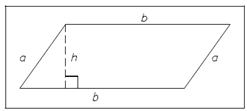## Quadrilaterals Assignment Help

Assignment Help: >> Shapes and Figures of Plane Geometry - Quadrilaterals

Quadrilateral is any four-sided geometric figure.Figure: Parallelogram

The diagram which is given above is parallelogram which has four-sided quadrilateral with both pairs of opposite side's parallel, as shown in above figure.

The area of the parallelogram is calculated using the following formula:

A = (base) • (height) = bh

The perimeter of a parallelogram is computed using the subsequent formula:

P = 2a + 2b

An  area  of  a  parallelogram  is  always  expressed  in  square  units,  and  the  perimeter  of  a parallelogram is always expressed in the original units.#### Assured A++ Grade

Get guaranteed satisfaction & time on delivery in every assignment order you paid with us! We ensure premium quality solution document along with free turntin report!